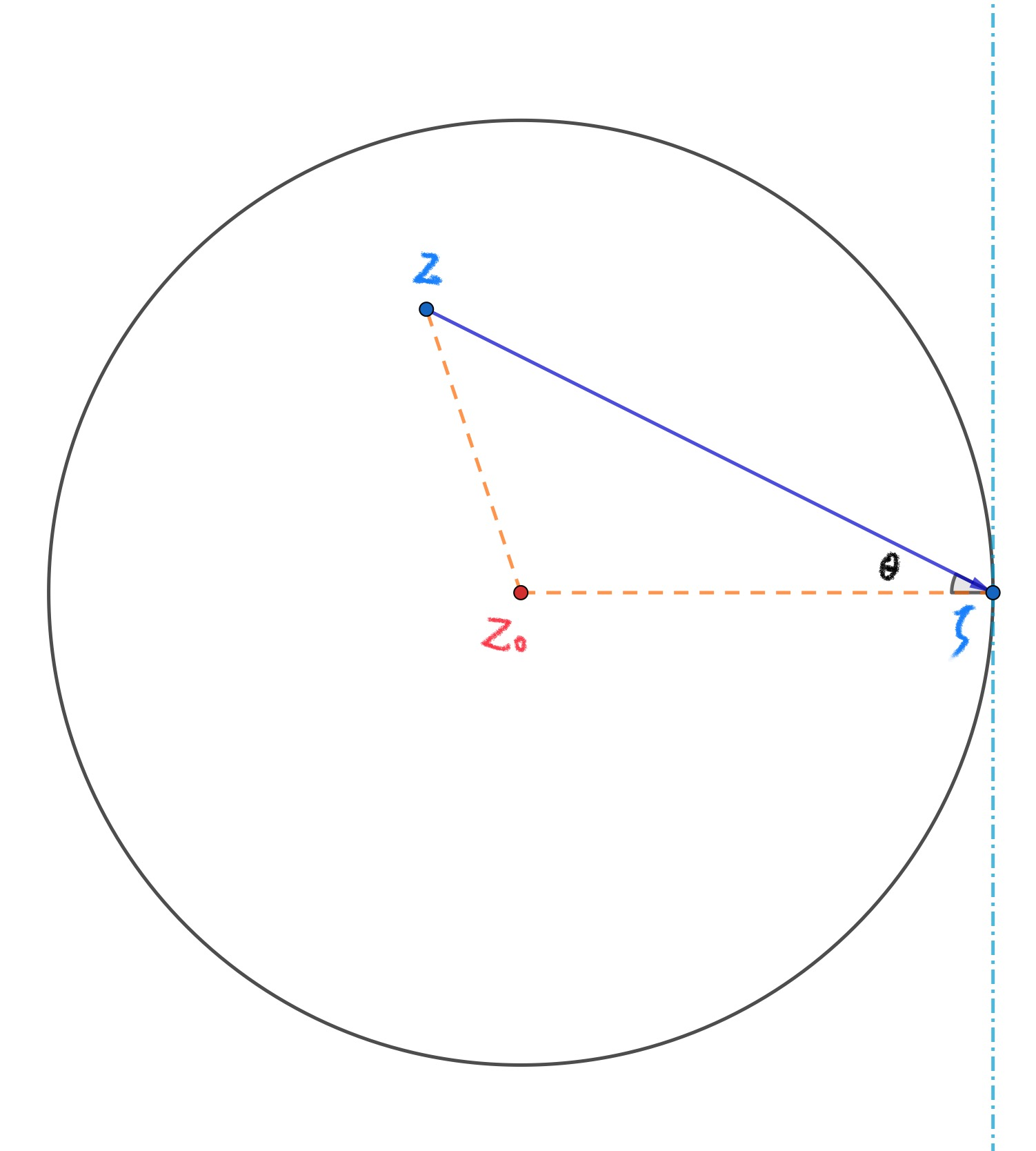# 数学分析习题解-一致收敛性、函数项级数与函数族的基本运算(2)

【习题来源】数学分析：第七版.（俄罗斯）卓里奇著；李植译. 北京：高等教育出版社，2019.2

1. 请研究下列级数当实参数 $\alpha$ 取各种值时在集合 $E\subset\mathbb{R}$ 上的收敛性.
a) $$\sum_{n=1}^{\infty}\frac{\cos nx}{n^\alpha};$$
b) $$\sum_{n=1}^{\infty}\frac{\sin nx}{n^\alpha}.$$

【解】 显然, 上述两个级数在 $\alpha\leqslant 0$ 时不收敛, 在 $\alpha > 1$ 时绝对一致收敛(强函数检验法). 考虑在 $0< \alpha \leqslant 1$ 时的情况.

$$\begin{array}{c}\sum_{n=1}^{\infty}\cos nx=\frac{\cos\frac{n}{2}x \cdot \sin\frac{n+1}{2}x}{\sin\frac{x}{2}}\\ \sum_{n=1}^{\infty}\sin nx=\frac{\sin\frac{n}{2}x \cdot \sin\frac{n+1}{2}x}{\sin\frac{x}{2}}\end{array}$$

【幂级数的阿贝尔第二定理】 如果幂级数 $\sum_{n=1}^{\infty}c_n(z-z_0)^n$ 在某点 $\zeta\in \mathbb{C}$ 收敛, 则它在以 $z_0$, $\zeta$ 为端点的闭区间上一致收敛.

1. (阿贝尔第二定理的补充) 证明: 如果一个幂级数在其收敛圆边界上的某一个点收敛, 则它的和在这个圆中沿着任何不与圆周相切的方向趋于该点时均有极限.

【证明】 由于幂级数 $\sum_{n=1}^{\infty}c_n(z-z_0)^n$ 的收敛圆以 $z_0$ 为圆心, $r = 1/\overline{\lim_{n\rightarrow\infty}}\sqrt[n]{|c_n|}$ 为半径. 为简明记, 不失一般性的, 设 $z_0 = 0$, 收敛半径为 $1$. 再设幂级数在收敛圆边界上的一点 $\zeta = 1$ 处收敛 ( 对于一般形式的幂级数, 在边界上一点收敛, 设 $\widetilde{z}= (z - z_0)/(\widetilde{\zeta} - z_0)$, 令 $\widetilde{c}_n = c_n(\zeta - z_0)^n$ 即化为我们所做的简化假设的形式 $\sum \widetilde{c}_n \widetilde{z}^{n}$ ).

$$\begin{equation}\label{eq:1} \left|\sum_{k=n+1}^{m}c_k z^k\right| \end{equation}$$

$$\begin{equation}\label{eq:2}|s_m-s_n| = \left|\sum_{k=n+1}^{m}c_k\right| < \varepsilon.\end{equation}$$

$$\begin{array}{cl} \left|\sum_{k=n+1}^{m}c_k z^k\right| &=& \left|\sum_{k=n+1}^{m}(A_k - A_{k-1}) z^k\right|\\ &=& \left|A_m z^m - A_n z^{n+1} + \sum_{k=n+1}^{m-1}A_k(z^k - z^{k+1})\right|\\ &\leqslant& \left|A_m z^m - A_n z^{n+1}\right| + \left|\sum_{k=n+1}^{m-1}A_k(z^k - z^{k+1})\right|\\ &\leqslant& \left|A_m - A_n \right||z^m| + \sum_{k=n+1}^{m-1}\left|A_k(z^k - z^{k+1})\right|\\ &\leqslant& \left|A_m - A_n \right||z^m| + |1-z|\sum_{k=n+1}^{m-1}\left|A_kz^k\right| \end{array}$$

$$\begin{equation}\label{eq:3} \begin{array}{cl} \left|\sum_{k=n+1}^{m}c_k z^k\right| &\leqslant& \varepsilon + \varepsilon\cdot|1-z|\sum_{k=n+1}^{m-1}\left|z^k\right|\\ &<& \varepsilon + \varepsilon\cdot\frac{|1-z|}{1-|z|}.\quad(\text{用到等比数列求和}) \end{array} \end{equation}$$

$$|\zeta-z|^2 + |\zeta - z_0|^2 -2|\zeta-z||\zeta-z_0|\cos\theta = |z-z_0|^2$$

$$\begin{equation}\label{eq:4} |1-z|^2 + 1 -2|1-z|\cos\theta = |z|^2. \end{equation}$$

$$\frac{|1-z|}{1-|z|} = \frac{1+|z|}{2\cos\theta-|1-z|},$$
$z$ 趋于并足够接近 $1$ 时, 总有 $|1-\zeta|<\cos\theta$ (因为 $0 < \theta < 1$). 故而, 对式 (\ref{eq:3}) 进一步估计得到
$$\left|\sum_{k=n+1}^{m}c_k z^k\right| < \varepsilon + \varepsilon\cdot\frac{2}{\cos\theta}.$$https://zengfk.com.cn/2019/10/22/数学分析习题解-一致收敛性、函数项级数与函数族的基本运算-2/

Zengfk

2019-10-22

2019-10-23